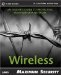# Public Key Cryptography

In public key cryptography, the encryption and decryption keys are different. This is in contrast to symmetric key cryptography, where a single key is used for both encryption and decryption. In public key encryption, each user has a pair of keys, known as a public key and a private key . Public key encryption utilizes a one-way function to scramble data using the recipient's public key . The recipient must then use his private key to decrypt the data.

#### Common Public Key Algorithms

In encryption applications, a tested and proven mathematical algorithm is almost always the strongest link in the security chain. Instead, hackers find other chinks in the armor , usually by exploiting weaknesses in the software packages (reverse engineering) or in their human operators (social engineering). Thus, for most purposes, the encryption algorithm that you choose is actually the least important part.

However, advances in the field of quantum cryptography might soon reverse the situation. Using the semi-infinite power of molecular computing, it might soon be possible to effortlessly break encryption of any conceivable strength. For now, though, most algorithms are suitably strong. The following are some of the most commonly used and tested public-key algorithms:

• RSA ” The algorithm is named after its three inventors: Ron Rivest, Adi Shamir, and Leonard Adleman. It is currently the most commonly used public key algorithm. RSA is cryptographically strong, and is based on the difficulty of factoring large numbers . RSA is also unique in that it is capable of both digital signature and key exchange operations.

• DSA ” The United States National Security Agency (NSA) invented DSA (Digital Signature Algorithm). This algorithm can be used for digital signature operations, but not for data encryption. Its cryptographic strength is based on the difficulty of calculating discrete logarithms.

• Diffie-Hellman ” Diffie-Hellman was named after its inventors Whitfield Diffie and Martin Hellman. This algorithm can be used for key exchange only. The cryptographic strength of Diffie-Hellman is based on the difficulty of calculating discrete logarithms in a finite field.Maximum Wireless Security
ISBN: 0672324881
EAN: 2147483647
Year: 2002
Pages: 171

Similar book on Amazon